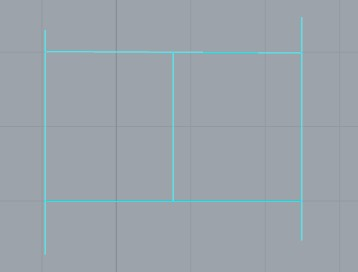# Line grouping to direction

Hello people!

I have an initial group of lines that I split all together with a karamba component but now the lines= beams of all directions are in the same list. How can I list lines according to direction. Now my direction is x and y but I want to apply this code to 3d directions. Any suggestions?

Thanks!
PopiYou can measure the tangents of each line, convert those vectors to points, and then group those points by proximity: direction sort.gh (19.5 KB)

1 Like

Thank you for the quick answer!
I tried it and it worked, but I want to use it on a 3d shape that the UV cordinates have been converted in polylines and are used as beams, so the directions of the lines should follow the ones of UV. Could you explain a bit more the part of “convert tangents to point”? I did not understand how you managed to group them.

Thank you!

This here works because lines have the same tangent vector everywhere. If you’re dealing with curved splines, then you’ll have to compute some sort of average tangent, or maybe just look at the start-end vector.

A vector (in 3d-space) is an ordered triplet of numbers. One telling you the amount this vector travels along the world X axis, one for y and one for z. You can write a vector like (x,y,z). Points (in 3d-space) are also ordered triplets of numbers, although in this case they are coordinates rather than factors.

Since vectors are points are both triplets, they are easy to convert into each other, even though the meaning of the numbers is different. Grasshopper doesn’t have a component for grouping similar vectors, but it does have one for grouping similar points, so that’s why we have to convert the data from one type (vectors) into another (points).

The Point Group component uses a distance metric to figure out which points in a cloud would belong to the same group. This is a bit awkward since what we really want is an angle metric between vector directions rather than a distance metric between points. And if you need exact control over angles we’ll have to do some trig to figure out a conversion between those. However you can probably just pick the threshold with some manual fiddling until you have a value that works.

maybe I have to approach this problem differently. My lines initialy in x direction are in one list and in y in a different. Also, the initial lines get split in smaller segments. Could make again 2 lists or branges based which line they initially got splitted from?

Maybe a code for overlaping main curve with sub curves would do. Do you know if this is possible?

Hi @DavidRutten,
How can i make this technique work for more then just one input list. (see example below)
It looks like some branches are doubled and some ar ignored.